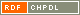# Show document

Title: Isomorphism checking of I-graphs Horvat, Boris (Author)Pisanski, Tomaž (Author)Žitnik, Arjana (Author)http://dx.doi.org/10.1007/s00373-011-1086-2 English Not categorized 1.01 - Original Scientific Article IAM - Andrej Marušič Institute We consider the class of ▫$I$▫-graphs, which is a generalization of the class of the generalized Petersen graphs. We show that two ▫$I$▫-graphs ▫$I(n, j, k)$▫ and ▫$I(n, j_1, k_1)$▫ are isomorphic if and only if there exists an integer ▫$a$▫ relatively prime to $n$ such that either ▫$\{j_1, k_1\} = \{aj \mod n, \; ak \mod n \}$▫ or ▫$\{j_1, k_1\} = \{aj \mod n, \; -ak \mod n\}$▫. This result has an application in the enumeration of non-isomorphic ▫$I$▫-graphs and unit-distance representations of generalized Petersen graphs. mathematics, graph theory, isomorphism, I-graph, generalized Petersen graph 2012 str. 823-830 Vol. 28, no. 6 0911-0119 519.17 160699771601 76Document is not linked to any category.

Average score: (0 votes) Voting is allowed only to logged in users.Hover the mouse pointer over a document title to show the abstract or click on the title to get all document metadata.

## Secondary language

Language: Slovenian Obravnavamo razred ▫$I$▫-grafov - posplošitev razreda t.i. posplošenih Petersenovih grafov. Pokažemo da sta ▫$I$▫-grafa ▫$I(n, j, k)$▫ in ▫$I(n, j_1, k_1)$▫ izomorfna natanko takrat, ko obstaja celo število ▫$a$▫ tuje z ▫$n$▫, za katerega velja, da drži bodisi ▫$\{j_1, k_1\} = \{aj \mod n, \; ak \mod n \}$▫ bodisi ▫$\{j_1, k_1\} = \{aj \mod n, \; -ak \mod n\}$▫. Ta rezultat je uporaben pri preštevanju neizomorfnih ▫$I$▫-grafov in predstavitev z enotsko razdaljo posplošenih Petersenovih grafov. matematika, teorija grafov, izomorfizem, I-graf, posplošeni Petersenov graf